## Maclaurin TrisectrixA curve first studied by Colin Maclaurinin 1742. It was studied to provide a solution to one of the Geometric Problems of Antiquity, in particular Trisection of an Angle, whence the name trisectrix. The Maclaurin trisectrix is an Anallagmatic Curve, and the origin is a Crunode.

The Maclaurin trisectrix has Cartesian equation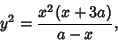(1)

or the parametric equations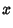(2)(3)

The Asymptote has equation, and the center of the loop is at. If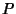is a point on the loop so that the line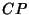makes an Angle of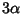with the negative y-Axis, then the line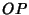will make an Angle ofwith the negative y-Axis.

The Maclaurin trisectrix is sometimes defined instead as(4)(5)(6)

Another form of the equation is the Polar Equation(7)

where the origin is inside the loop and the crossing point is on the Negative x-Axis.

The tangents to the curve at the origin make angles ofwith the x-Axis. The Area of the loop is(8)

and the Negative-intercept is(MacTutor Archive).

The Maclaurin trisectrix is the Pedal Curve of the Parabola where the Pedal Point is taken as the reflection of the Focus in the Directrix.

References

Lawrence, J. D. A Catalog of Special Plane Curves. New York: Dover, pp. 103-106, 1972.

Lee, X. Trisectrix.'' http://www.best.com/~xah/SpecialPlaneCurves_dir/Trisectrix_dir/trisectrix.html.

Lee, X. Trisectrix of Maclaurin.'' http://www.best.com/~xah/SpecialPlaneCurves_dir/TriOfMaclaurin_dir/triOfMaclaurin.html

MacTutor History of Mathematics Archive. Trisectrix of Maclaurin.'' http://www-groups.dcs.st-and.ac.uk/~history/Curves/Trisectrix.html.